• 分支结构分支结构分支结构 更方便的是！！ 分支结构——嵌套if的使用 条件表达式 （用加号改成字符串的连接 pass语句 注释：对象bool值什么时候是false：
单分支结构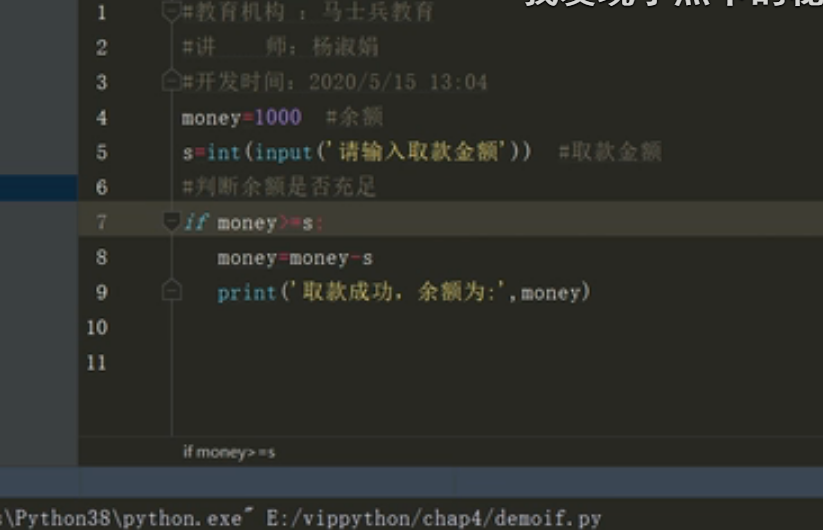双分支结构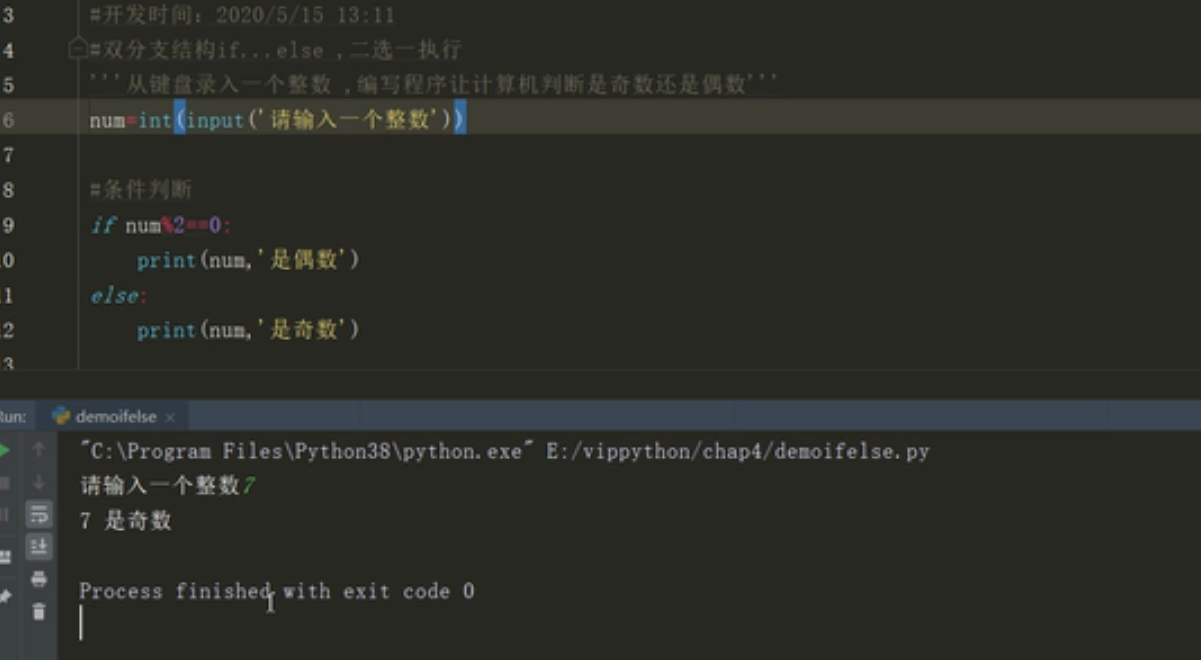多分支结构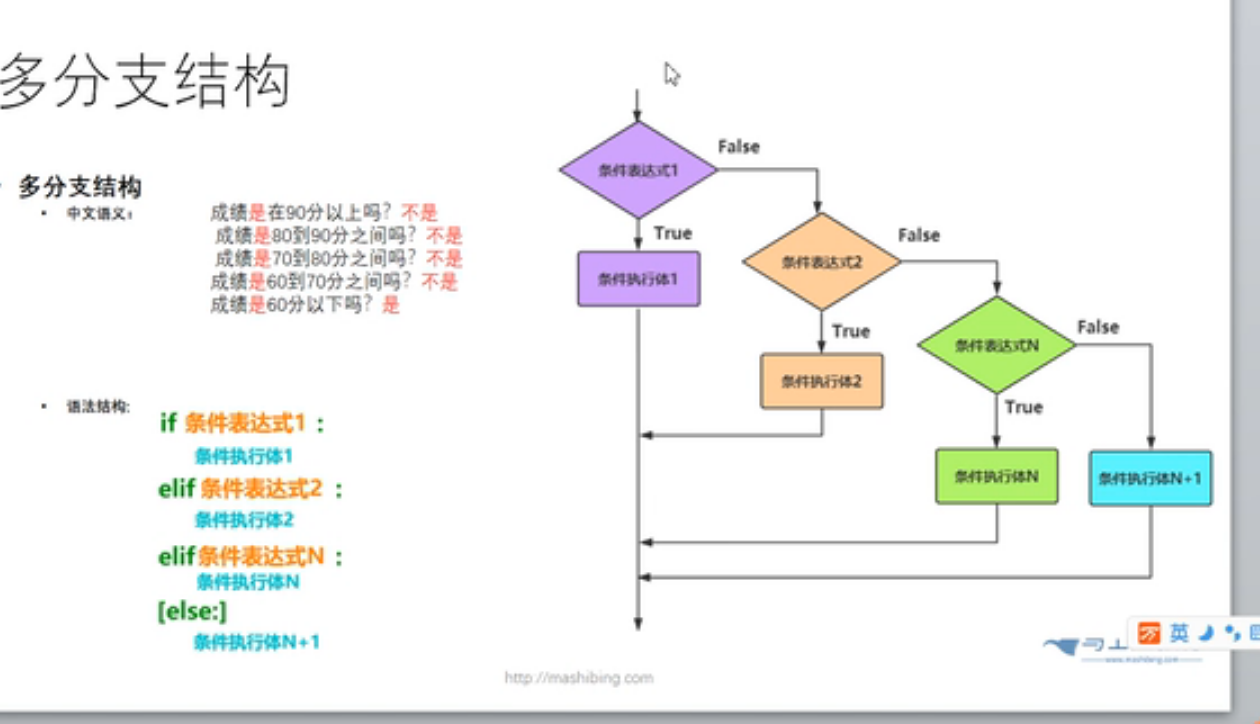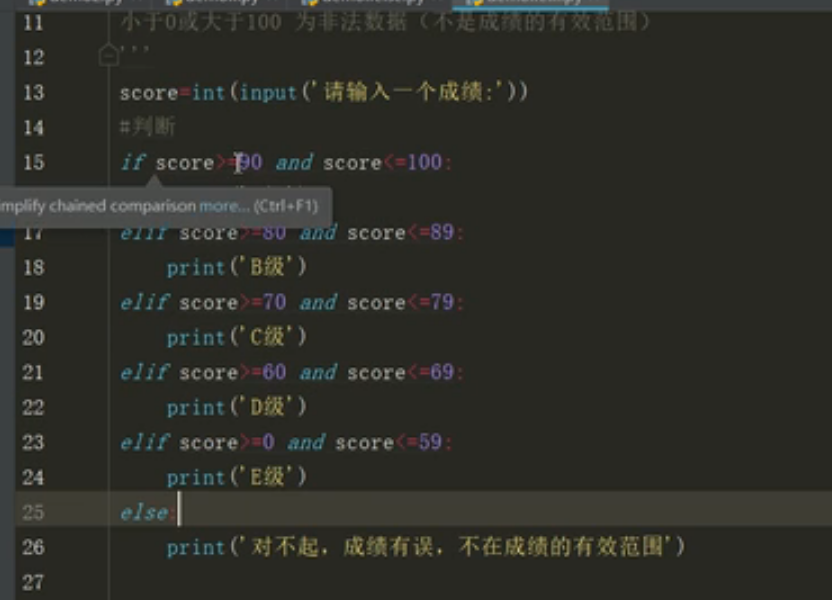更方便的是！！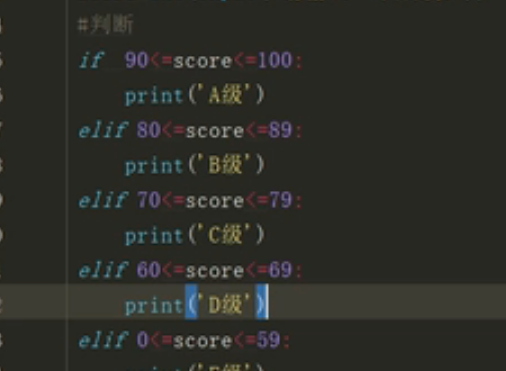分支结构——嵌套if的使用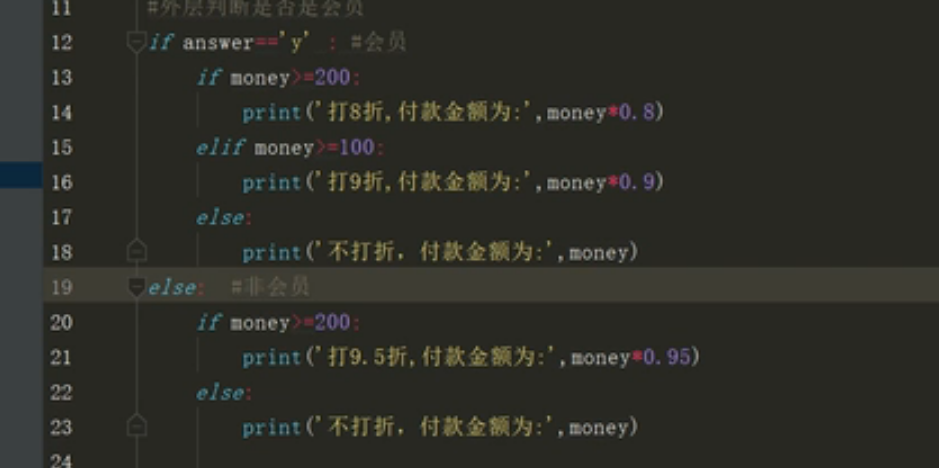条件表达式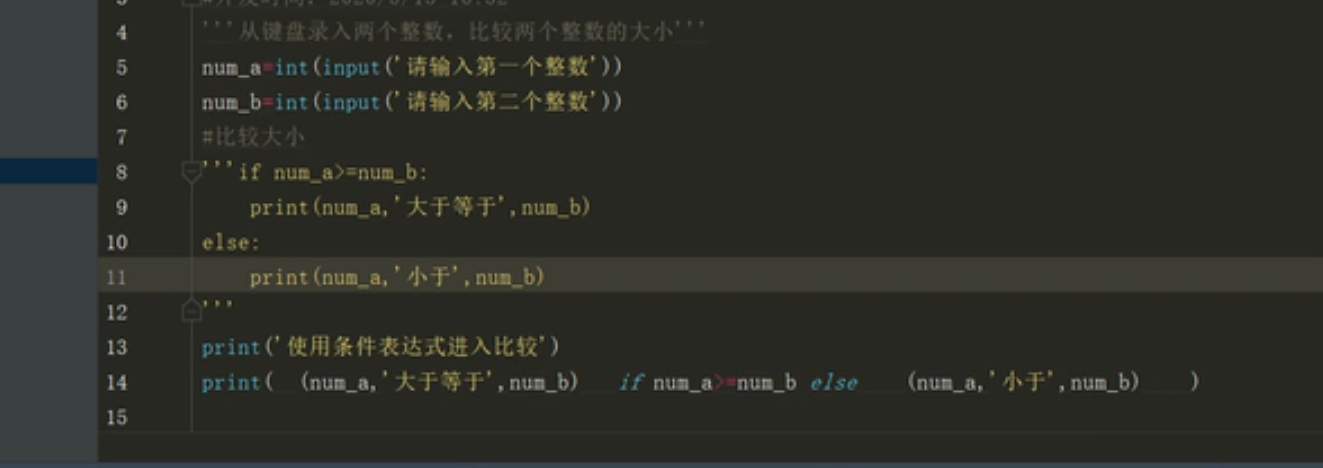（用加号改成字符串的连接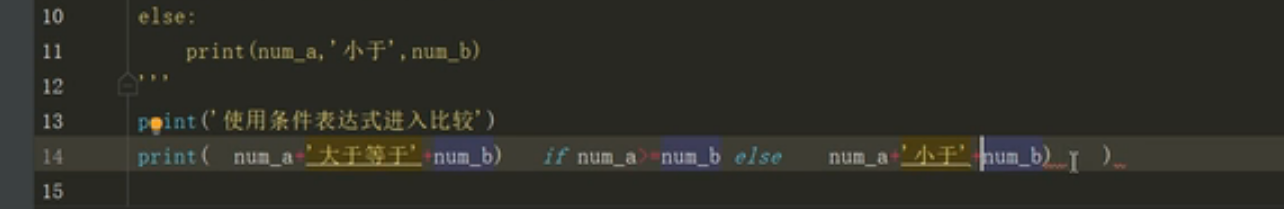pass语句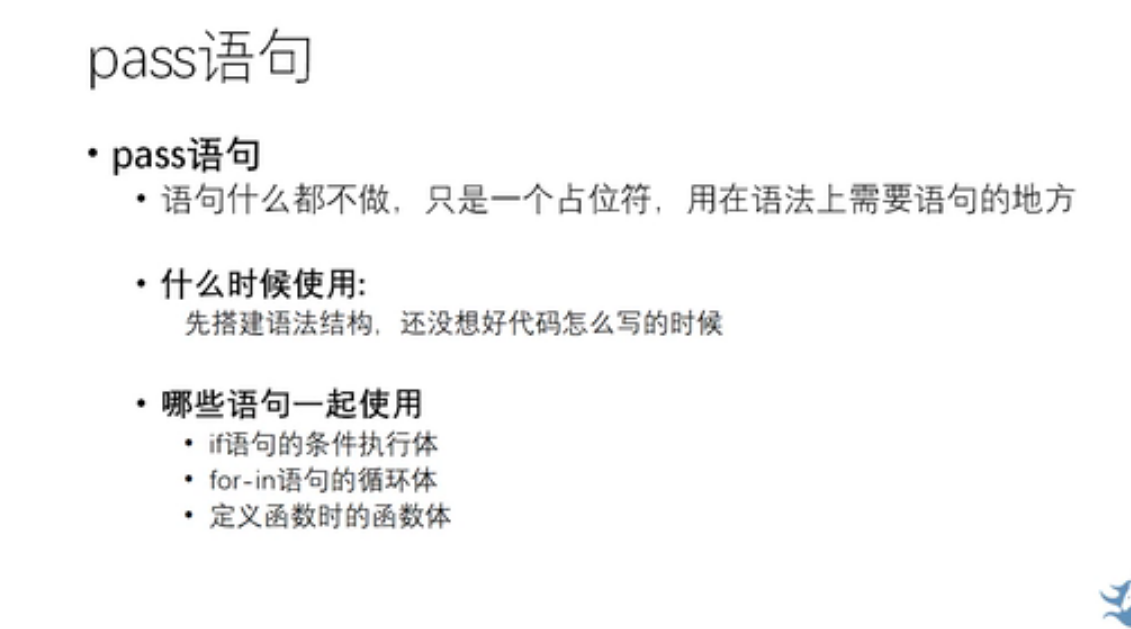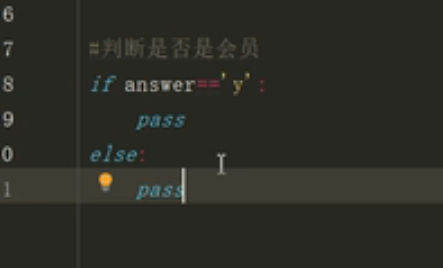注释：对象bool值什么时候是false：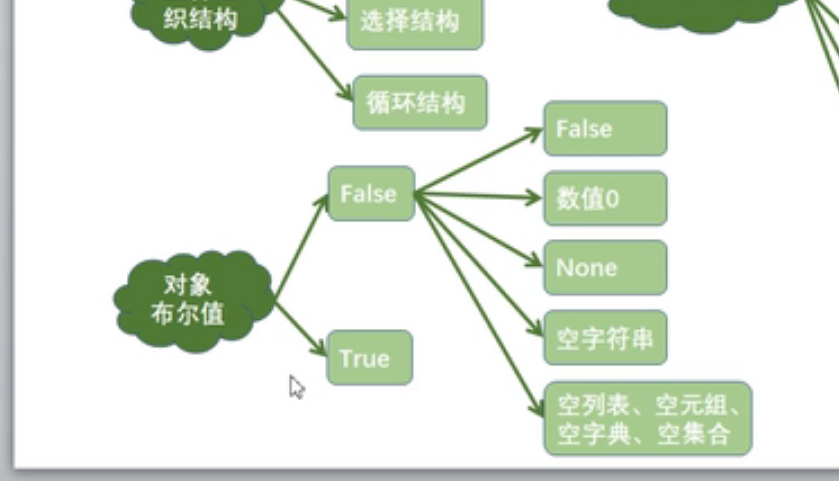展开全文python
• 一、MATLAB 流程控制结构、 二、for 循环、 1、for 循环、 2、嵌套 for 循环、 三、while 循环、 二、分支结构、 1、if end 分支结构、 2、if else end 分支结构、 3、switch case end 分支结构
文章目录一、MATLAB 流程控制结构二、for 循环1、for 循环2、嵌套 for 循环三、while 循环二、分支结构1、if end 分支结构2、if else end 分支结构3、switch case end 分支结构

一、MATLAB 流程控制结构

MATLAB 流程控制结构 :
顺序结构 : 默认从上到下执行 ;
分支结构 :

if else end
switch case end

循环结构 :

for end
while end

二、for 循环

1、for 循环

for 循环语法 : 如果步长为 $1$ , 可以省略 ;
for 循环变量 = 初值 : 步长 : 终值

执行语句

end


for 循环示例 : 求  $1^2 + 2^2 +3^2$ 的和 ;
这里设置一个 $1$  ~ $3$ 的循环 , 步长为 $1$ , 因此中间的步长参数 , 可以省略 ;

代码示例 :
%% for 循环

% for 循环
% 定义结果存储的变量 , 注意要赋值一个初始值
sum = 0;

% 循环控制变量 i
% 从 1 循环到 3 , 步长为 1 (可省略)
for i = 1:1:3

% 循环体操作
sum = sum + i^2;

% 循环结束标志
end

% 获取 sum 值
sum


执行结果 : 点击 " 运行 " 按钮 , 运行该脚本 , 在命令行窗口输出 sum 结果 , $14$ ;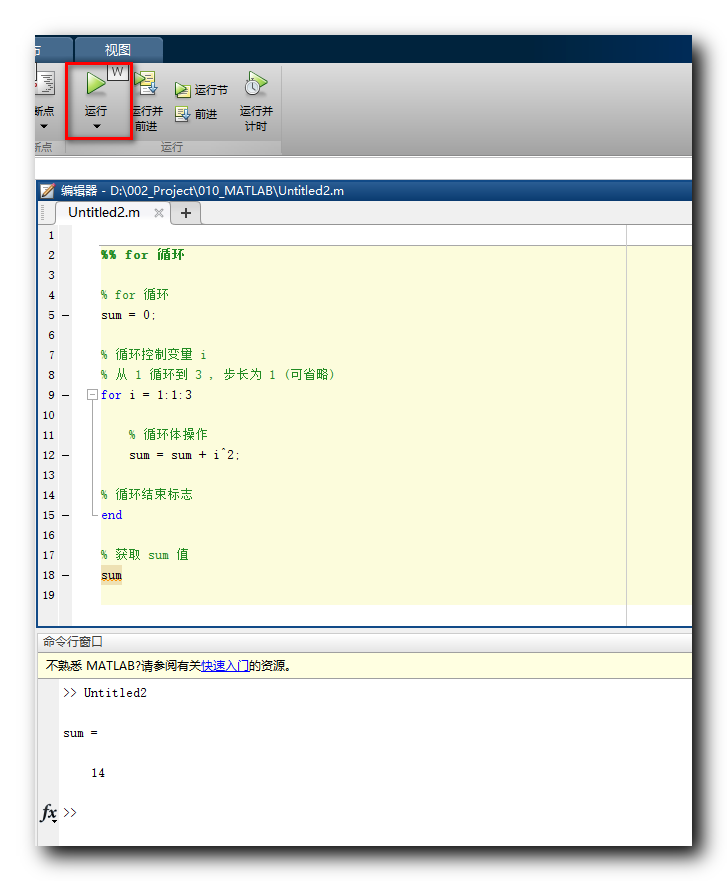2、嵌套 for 循环

使用 for 循环 , 求 $\rm \sum_{i = 1}^{5} i!$ ;

将 $\rm \sum_{i = 1}^{5} i!$ 式子展开 :
$\rm \sum_{i = 1}^{5} i! = 1! + 2! + 3! + 4! + 5!$

阶乘运算 : $5! = 5 \times 4 \times 3 \times 2 \times 1$

程序设计 : 外层循环循环 $5$ 次 , 内层循环分别循环 $1, 2, 3 , 4, 5$ 次 ;

代码示例 :
%% 嵌套 for 循环

% for 循环
% 定义结果存储的变量 , 注意要赋值一个初始值
sum = 0;

for i = 1:1:5

% 内层循环中求阶乘
sum_in = 1;

% 求 i 的阶乘
for j = 1:1:i
sum_in = sum_in * j;
end

% 累加和
sum = sum + sum_in;

end

% 最终阶乘累加结果
sum


执行结果 :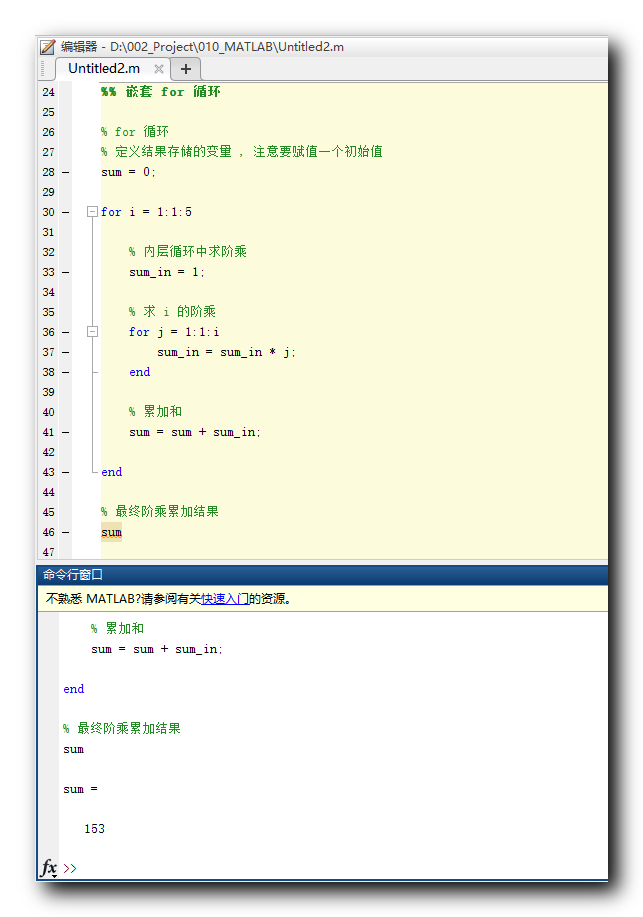三、while 循环

while 循环语法 : 如果条件表达式成立 , 执行循环 , 如果不成立 , 跳出循环 ;
while 条件表达式

// 执行语句

end


while 循环示例  :
求 $1 +2 + 3+ \cdots + 10$ 之和 ;

代码示例 :
%% while 循环

% 存放累加和的变量
sum = 0;
% 循环控制变量
i = 0;

while i < 11
% 累加操作
sum = sum + i;
% 循环控制变量改变
i = i + 1;
end

% 最终累加结果
sum


执行结果 :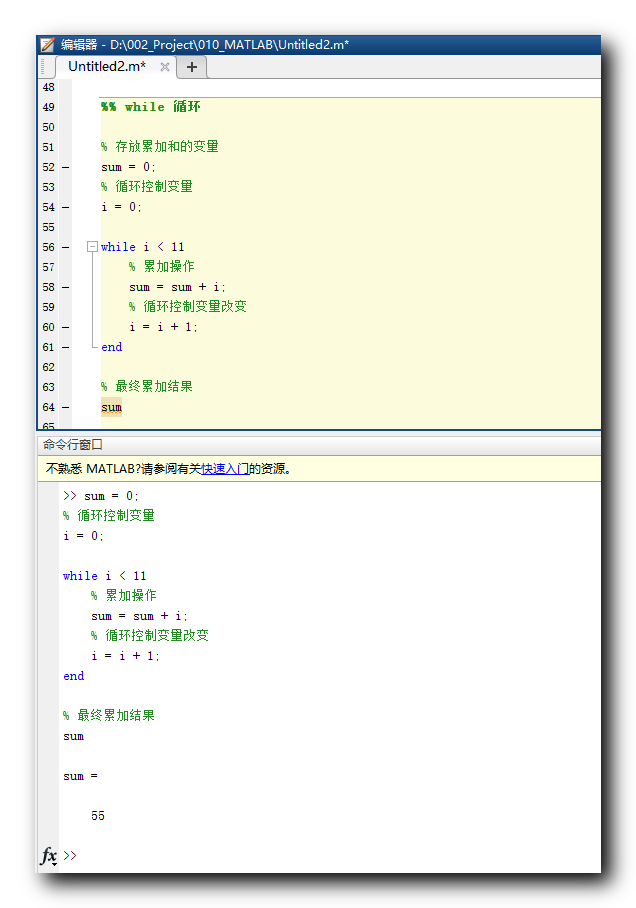二、分支结构

1、if end 分支结构

if end 分支结构语法 : 条件表达式成立 , 执行 " 执行语句 " , 不成立 , 直接跳出到 end ;
if 条件表达式
// 执行语句
end


代码示例 :
%% if end 分支结构

score = 61

if score > 60
'及格'
end


执行结果 :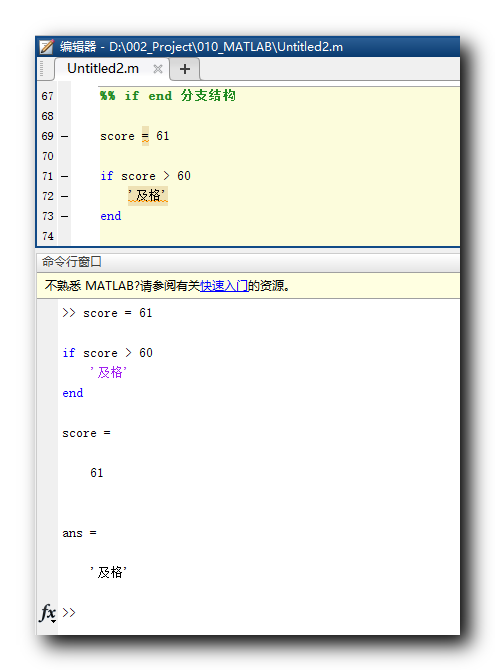2、if else end 分支结构

if else end 分支结构语法 : 如果 条件表达式 成立 , 执行 " 执行语句1 " , 否则执行 " 执行语句2 " ;
if 条件表达式
// 执行语句 1
else
// 执行语句 2
end


代码示例 :
%% if else end 分支结构

score = 61

if score > 60
'及格'
else
'不及格'
end


执行结果 :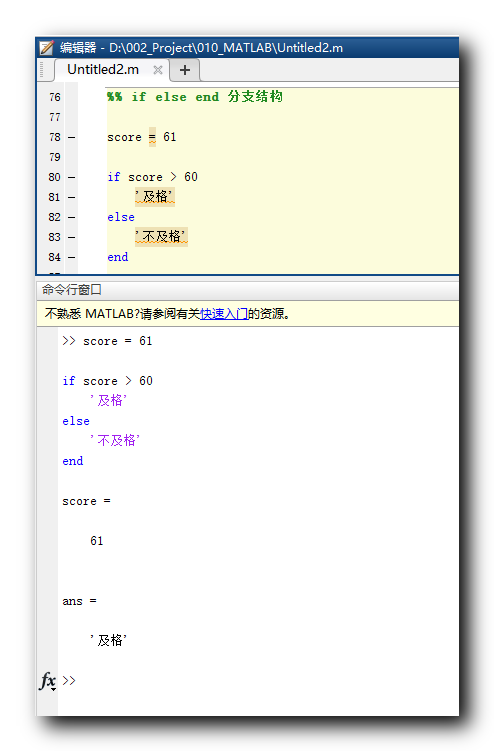3、switch case end 分支结构

switch case end 分支结构语法 : 通过表达式的值进行比较 , 通过不同的比较结果 , 实现分支功能 ; 如果所有语句都不满足 , 跳转到 otherwise 分支 , 如果没有定义 otherwise 分支 , 则直接跳出到 end ;
switch 表达式
case 表达式1
执行语句1
case 表达式2
执行语句2
.
.
.
otherwise
执行语句n
end


代码示例 :
%% switch case end 分支结构

score = 100

switch score
case 60
'及格'
case 100
'满分'
otherwise
'其它分数'
end


执行结果 :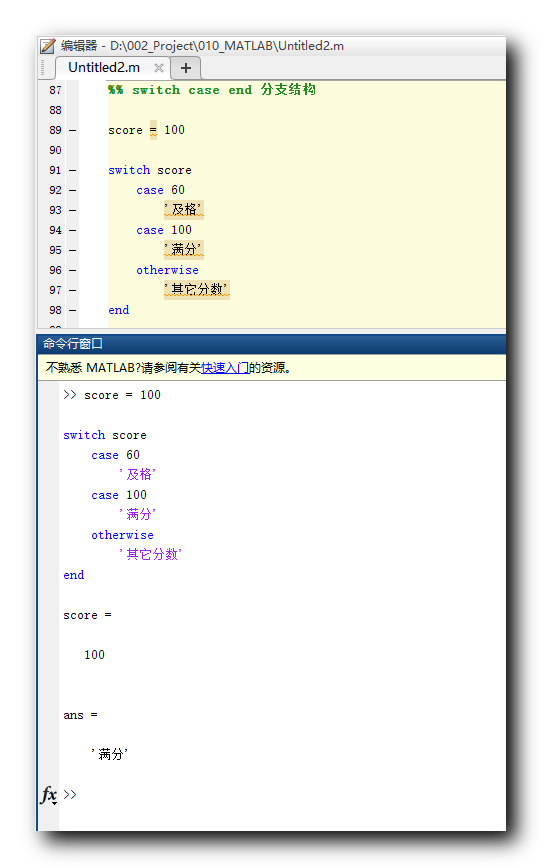展开全文matlab
• 分支结构程序 分支结构程序 分支结构程序 分支结构程序
• 分支结构 switch
1 分支结构
1.1 概述
顺序结构的程序虽然能解决计算、输出等问题
但不能做判断再选择。对于要先做判断再选择的问题就要使用分支结构
1.2 形式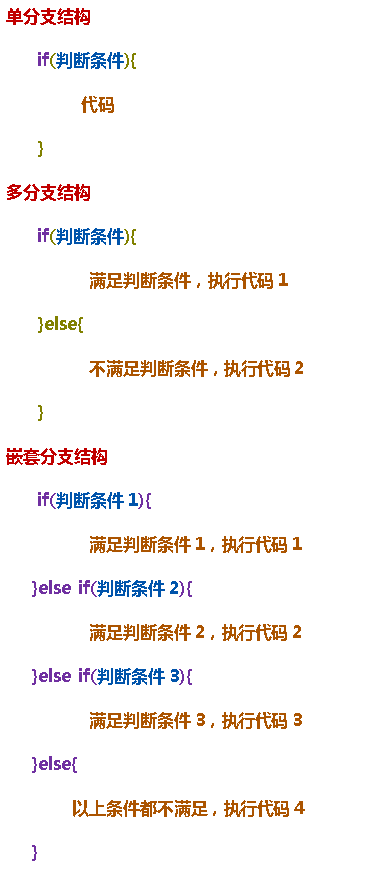1.3.1 练习：商品打折案例
创建包: cn.tedu.basic
创建类: TestDiscount.java
需求: 接收用户输入的原价。满1000打9折;满2000打8折;满5000打5折
package cn.tedu.basic;

import java.util.Scanner;

/**需求：接收用户输入的原价，满1000打9折，满2000打8折,满5000打5折*/
public class TestDiscount {
public static void main(String[] args) {
//1.提示用户输入原价
System.out.println("请输入商品原价:");
//2.接收用户输入的原价
double price = new Scanner(System.in).nextDouble();
//3.计算打折后的价格
//3.1定义变量用来保存打折后的价格
double count = price;//初始值是商品的原价
//3.2判断用户的打折段位并打折
if(price >= 5000) {//满5000
count = price *0.5;//打5折
}else if(price >= 2000) {//满2000
count = price * 0.8;//打折8折
}else if(price >= 1000) {//满1000
count = price *0.9;//打9折
}
//		if(1000 <= price && price< 2000) {
//			count = price *0.9;
//		}else if(2000 <= price && price < 5000) {
//			count = price * 0.8;
//		}else if(price >= 5000) {
//			count = price *0.5;
//		}
//3.3输出用户实际支付的价格
System.out.println("您实际应该支付:"+count);
}
}

1.3.2 练习: 统计学员得分段位案例
package cn.tedu.basic;

import java.util.Scanner;

/*本类用于判断学员分数的档位*/
public class TestScore {
public static void main(String[] args) {
//1.提示并接收学员的分数
System.out.println("请输入你的分数：");
int score = new Scanner(System.in).nextInt();
/*90分及以上  优秀
*80-89 良好
*70-79 中等
*60-69 及格
*60分及以下 不及格 */

/**为了增强程序的健壮性，如果用户输入的数据不符合规则，就结束*/
if(score <0 || score >100 ) {
System.out.println("输入数据不合法，请重新输入！！！");
}else {
//2.判断分数的段位，并输出结果
if(score >= 90) {//大于90
System.out.println("优秀！");
//}else if(score>=80 && score <=89) {
}else if(score>=80) {//[80,90)
System.out.println("良好！");
}else if(score>=70) {//[70,80)
System.out.println("中等！");
}else if(score>=60) {//[60,70)
System.out.println("及格！");
}else {//小于60
System.out.println("不及格！");
}
}
}
}


2 switch结构
2.1 概述
switch case 语句用来判断一个变量与一系列值中某个值是否相等，每个值称为一个分支。
当一个case成立，从这个case向后穿透所有case，包括default，直到程序结束或者遇到break程序才结束
2.2  形式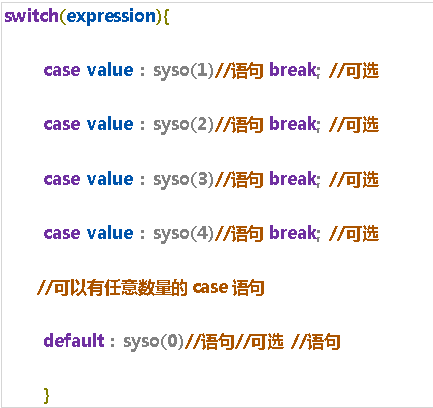2.3  练习：数字匹配
创建包: cn.tedu.basic
创建类: TestSwitch.java
package cn.tedu.basic;
/*本类用于练习switch结构*/
/**总结：
* 1.变量a的类型byte short char int String
* 2.会拿着变量a的值依次与case后的值做比较，如果不加break,
*        会向后穿透所有case,包括default
* 3.如果设置了default“保底选项”，并且没有任何case匹配到的话，就执行default
* 4.break与default是可选项，根据具体业务来决定加不加
* */
public class TestSwitch {
//1.创建程序的入口函数main
public static void main(String[] args) {
//2.定义一个变量
int a = 19;
//3.完成switch结构的测试
switch(a) {
case 1 : System.out.println(1);break;
case 2 : System.out.println(2);break;
case 3 : System.out.println(3);break;
case 4 : System.out.println(4);break;
case 5 : System.out.println(5);break;
default : System.out.println(0);
}
}

}


2.4 练习: Switch中的String类型
使用类: TestSwitch.java
public class TestSwitch {
public static void main(String[] args) {

String s = "Friday";
switch(s) {
case "Monday" : System.out.println("星期一吃火锅");break;
case "Tuesday" : System.out.println("星期二吃烤肉");break;
case "Wednesday" : System.out.println("星期三吃面条");break;
case "Thursday" : System.out.println("星期四吃小龙虾");break;
case "Friday" : System.out.println("星期五吃冒菜");break;
case "Saturaday" : System.out.println("星期六吃减脂餐");break;
case "Sunday" : System.out.println("星期日吃海鲜粥");break;

default : System.out.println("想吃啥吃啥吧");
}
}
}

2.5 switch结构的注意事项

switch 语句中的变量类型可以是： byte、short、int 、char、String(jdk1.7以后支持)
switch 语句可以拥有多个 case 语句
每个 case 后面跟一个要比较的值和冒号,且此值的数据类型必须与变量的数据类型一致
当变量值与 case 语句值相等时，开始执行此case 语句的内容,执行完会判断此行代码是否有break,如果有,结束执行,如果没有,会继续向后执行穿透所有case,包括default
switch 语句可以包含一个 default 分支，该分支一般是写在switch 语句的最后
如果在default之前的case有break,则default不会执行

3  循环结构
3.1  for概述
循环结构是指在程序中需要反复执行某个功能而设置的一种程序结构。
它由循环体中的条件，判断继续执行某个功能还是退出循环。
根据判断条件,循环结构又可细分为先判断后执行的循环结构和先执行后判断的循环结构。
3.2  for形式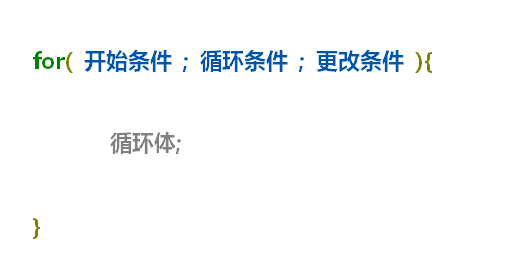3.3  for循环执行顺序
我们明明只写了一句打印语句,为什么打印了多个数字呢?
希望通过下图帮你理解for循环的执行顺序: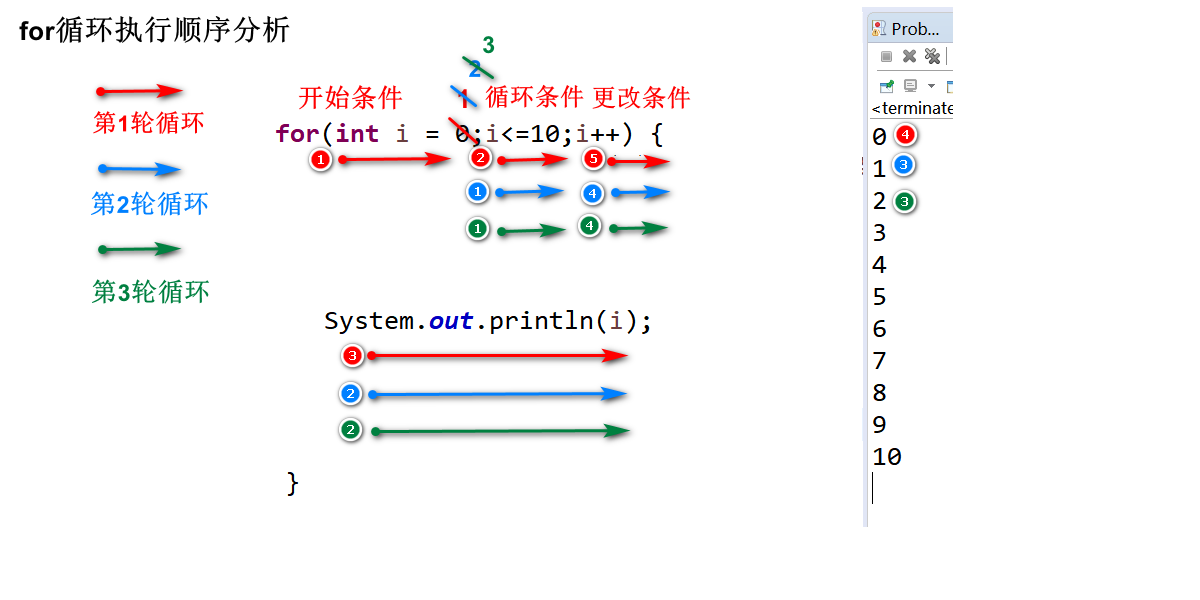3.4 练习：打印100次slogon/打印0到10/打印10到0/打印8,88,888,8888,
package cn.tedu.basic;
/**本类用来测试循环结构for循环*/
public class TestFor {
public static void main(String[] args) {
//需求:打印100次自己的宣言
//for(开始条件;循环条件;更改条件){循环体;}
for(int i = 1;i<=100;i++) {
System.out.println(“又不是没那条件，干就完啦！”);
}
//需求:打印0到10
//for(开始条件;循环条件;更改条件){循环体;}
//0 1 2 3 4 5 6 7 8 9 10
//从哪开始：0
//到哪结束：10
//怎么变化：+1 ++
for(int i = 0;i<=10;i++) {
System.out.println(i);
}
System.out.println("**********************");
//需求:打印10到0
//10 9 8 7 6 5 4 3 2 1 0
//从哪开始：10
//到哪结束：0
//怎么变化：-1  --
//for(开始条件;循环条件;更改条件){循环体}
for(int i = 10 ;i >= 0;i--) {
System.out.println(i);
}
//需求：打印8，88，888，8888,
//8 88 888 8888
//从何开始：8
//到哪结束：8888
//如何变化：*10+8
for(int i =8 ; i <= 8888 ; i=i*10+8) {
System.out.print(i+",");//使用的是print(),打印后不换行
}
}
}

3.5 练习:求[1,100]以内元素之和,偶数之和,偶数个数
package cn.tedu.basic;
/*本类用于测试for循环结构2*/
public class TestFor2 {
public static void main(String[] args) {
//m1();
//m2();
m3();
}
/*需求：求出1-100以内所有偶数的个数*/
private static void m3() {
//1.定义变量用来保存偶数的个数
int count = 0;
//2.创建循环，依次循环1-100范围内的数
for(int i = 1;i<101;i++) {
//3.过滤出来要统计个数的偶数
if(i % 2 ==0) {//说明这是一个偶数
//4.对偶数的个数进行累计
//count = count +1;
count++;
//++count;
}
}
//5.打印最终统计的个数
System.out.println(count);
}
/*需求：求出1-100以内所有偶数的和*/
private static void m2() {
//1.定义变量用来保存最终求和的结果
int sum = 0;
//2.创建循环，依次循环1-100范围内的数
for(int i = 1;i <101;i++) {
//3.过滤出来需要累加的偶数
if(i%2 == 0) {
//if(i%2 == 1) {//过滤奇数
//4.能进来，说明是偶数，累加
sum = sum +i;
}
}
//5.在for循环结束以后，打印所有偶数累加的和
System.out.println(sum);
}
/*需求：求出1-100以内所有数的和*/
private static void m1() {
//1.定义一个变量用来保存求和的结果
int sum = 0;
//2.创建循环，依次循环1-100范围内的数
for(int i = 1;i<=100;i++) {
//3.将本轮循环到的数字累加到最终的结果中
sum = sum + i;
}
//4.打印最终累加的结果
System.out.println(sum);
}
}



展开全文java if for循环 switch
• java分支结构题目java分支结构题目java分支结构题目java分支结构题目java分支结构题目java分支结构题目
• 分支结构 条件表达式详解 在选择和循环结构中，条件表达式为False的情况下： False/0/0.0/空值None、空序列对象（空序列、空字典、空元组、空集合、空字符串）、空range对象、空迭代对象 其它情况，均为True ...
控制语句

选择结构

单分支、双分支、多分支

单分支结构

条件表达式详解

在选择和循环结构中，条件表达式为False的情况下：

False/0/0.0/空值None、空序列对象（空序列、空字典、空元组、空集合、空字符串）、空range对象、空迭代对象

其它情况，均为True

条件表达式中，不能有赋值操作符“=”

双分支选择结构

三元运算符

多分支选择结构

选择结构的嵌套

注意控制好不同级别代码块的缩进量

#单分支结构
a = input("请输入一个小于10的数字：")if int(a)<10:
print(a)

#if 3<c and (c=20): 条件判断不能使用赋值操作符

b = [] #空列表

if b:

print("空的列表False")

if not b:

print("空的列表是True")

c = ""

if c:

print("c")

d = "False" #非空字符串

if d:

print("d")

e = 10

if e:

print("e")

#双分支结构

s = input("请输入一个数字：")

if int(s)<10:

print("s是小于10的数字")

else:

print("s是大于等于10的数字")

#三元条件运算符，简单的双分支赋值

#条件为真的值 if(条件表达式) else 条件为假的值

print("s是小于10的数字" if int(s)<10 else "s是大于等于10的数字")

score = int(input("请输入分数："))

grade = ""

if score<60:

grade="不及格"

elif score<80:  #60-80之间，大于等于60已经判断，没必要写

grade = "及格"

elif score<90:

grade = "良好"

else:

grade = "优秀"

print("分数是{0}，等级是{1}".format(score,grade))

if score<60:

grade="不及格"

elif 60<=score<80:

grade = "及格"

elif 80<=score<90:

grade = "良好"

elif 90<=score<=100:

grade = "优秀"

print("分数是{0}，等级是{1}".format(score,grade))

score = int(input("请输入分数："))

grade = ""

if score>100 or score <0:

score = int(input("输入错误，请重新输入分数："))

else:

if score>=90:

grade="A"

elif score>=80:

grade="B"

elif score>=70:

grade="C"

elif score>="60":

grade="D"

else:

grade="E"

print("分数为{0}，等级为{1}".format(score,grade))

#或者也可以用更少的代码

score = int(input("请输入一个分数"))

degree="ABCDE"

num=0

if score>100 or score<0:

print("请输入一个0-100的分数")

else:

num=score//10

if num<6:

num=5

print(degree[9-num])


展开全文• 分支结构分支结构单分支双分支多分支嵌套分支 分支结构 单分支 双分支 多分支 嵌套分支java
• 分支结构和循环结构 程序的三大控制结构为：顺序结构、循环结构、选择结构。 *分支结构其实就是选择结构 下面我介绍几种基本的分支结构： 1，if else语句 if else语句 又可以嵌套 嵌套分支语句虽可解决多个入口和...
• ## 1.5 Python分支结构

万次阅读 2019-05-18 12:11:59
1.5 Python分支结构1.5 Python分支结构:流程控制分支/选择结构(1)单项分支(2) 双项分支(3) 多项分支(4) 巢状分支 1.5 Python分支结构: 流程控制 流程: 计算机执行代码的顺序就是流程 流程控制: 对计算机...python 笔记 基础
• ## python分支结构

万次阅读 多人点赞 2019-05-10 20:49:09
分支结构 分支结构基本语法 if 条件表达式 语句一 语句二 语句三 ...... 条件表达式就是计算结果必须为布尔值的表达式 表达式后面的冒号不能少 注意if后面出现的语句，如果属于if...条件 循环 range函数 python
• 分支结构】 根据判断条件结果而选择不同向前路径的运行方式。 出现 if True: 则无条件执行后面的语句。 【 2.二分支结构】 紧凑型的二分支结构 适用于简单表达式的二分支结构 【 3.多分支结构】 【 4.......